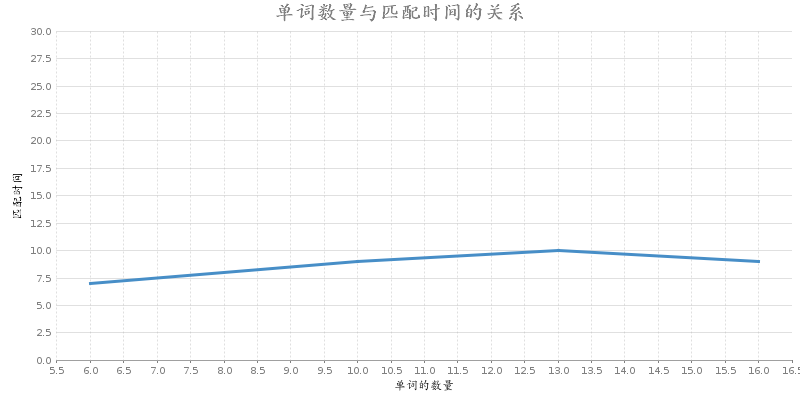AC算法的具体实现方法就是创建一棵前缀树，根据被查找的目标字符串，从树的根节点开始往叶子节点逐字符匹配。在这个过程中，如果发生失配，要根据失配跳转点进行跳转，如果找到匹配的模式串则进行打印输出。AC算法在扫描文本时完全不需要回溯，如果只考虑匹配的过程，该算法的时间复杂度为O(n)，也就是只跟待匹配文本的长度相关。 AC算法的实现可以由如下三个步骤构成： 1. 构造前缀树 2. 设置每个节点的失配跳转并收集每个节点的所有匹配模式串 3. 对目标字符串进行搜索匹配

### 步骤一：构造前缀树

``````begin
newstate ← 0
for i ← 1 util k do enter(yi)
for all a such that goto(0,a) == fail do goto(0,a) ← 0
end

procedure enter(a1a2…am):
begin
state ← 0
j ← 1
while goto(state, aj) ≠ fail do
begin
state ← goto(state, aj)
j ← j+1
end
for p ← j util m do
begin
newstate ← newstate + 1
goto(state, ap) ← newstate
state ← newstate
end
output(state) ← {a1a2…am}
end
``````

### 步骤二：设置每个节点的失配跳转

fail值None000120303

``````begin
queue ← empty
for each a such that goto(0,a) = s ≠ fail do
begin
queue ← queue U {s}
fail(s) ← 0
end
while queue ≠ empty do
begin
let r be the next state in queue
queue ← queue - {r}
for each a such that goto(r,a) = s ≠ fail do
begin
queue ← queue U {s}
state ← fail(r)
while goto(state,a) = fail do state ← fail(state)
fail(s) ← goto(state,a)
output(s) ← output(s) U output(fail(s))
end
end
end
``````

### 步骤三：对目标字符串进行搜索匹配

``````begin
state ← 0
for i ← 1 until n do
begin
while goto(state,ai) = fail do state = fail(state)
state ← goto(state,ai)
if output(state) ≠ empty then
begin
print output(state)
end
end
end
``````

``````public class Keyword implements Serializable {

private Integer id;

private Map<Integer, Integer> categoryTypeMap;

private String word;

private List<Integer> categories; //当前的关键词属于哪几个分类

getter and setter ...

@Override
public boolean equals(Object o) {
if (this == o) return true;
if (o == null || getClass() != o.getClass()) return false;

Keyword keyword = (Keyword) o;

if (id != null ? !id.equals(keyword.id) : keyword.id != null) return false;
return true;
}

@Override
public int hashCode() {
return id != null ? id.hashCode() : 0;
}

}
``````

``````static class Node{
int state;                    //自动机的状态，也就是节点数字
char character = 0;           //指向当前节点的字符，也即条件
Node failureNode;             //匹配失败时，下一个节点
List<Keyword> keywords;       //匹配成功时，当前节点对应的关键词
List<Node> children;          //当前节点的子节点
...
}
``````

``````public static class Patterns{

protected final Node root = new Node();
protected List<Node> tree;

public Patterns(List<Keyword> keywords) {
tree = new ArrayList<Node>();
for(Keyword keyword : keywords){
}
setFailNode();
}
public void addKeyword(Keyword keyword) {
char[] wordCharArr = keyword.getWord().toCharArray();
Node current = root;
for(char currentChar : wordCharArr){
if(current.containsChild(currentChar)){
current = current.getChild(currentChar);
} else {
Node node = new Node(table.size(), currentChar, root);
current = node;
}
}
}

public void setFailNode(){
Queue<Node> queue = new LinkedList<Node>();
Node node = root;
for (Node d1 : node.children)
queue.offer(d1);
while (!queue.isEmpty()) {
node = queue.poll();
if (node.children != null) {
for (Node curNode : node.children) {
queue.offer(curNode);
Node failNode = node.failureNode;
while (!failNode.containsChild(curNode.character)) {
failNode = failNode.failureNode;
if (failNode.state == 0) break;
}
if (failNode.containsChild(curNode.character)) {
curNode.failureNode = failNode.getChild(curNode.character);
}
}
}
}
}

}
``````

``````public Set<Keyword> searchKeyword(String data, Integer category) {
Set<Keyword> matchResult = new HashSet<Keyword>();
Node node = patterns.getRoot();
char[] chs = data.toCharArray();
for(int i=0; i < chs.length; i++){
while (!node.containsChild(chs[i])) {
node = node.failureNode;
if (node.state == 0) break;
}
if(node.containsChild(chs[i])){
node = node.getChild(chs[i]);
if(node.keywords != null){
for(Keyword pattern : node.keywords){
if (category == null) {
} else {
if (pattern.getCategories().contains(category)) {
}
}
}
}
}
}
return matchResult;
}
``````